# Reasoning, Justifying, Convincing and Proof

Reasoning, Justifying, Convincing and Proof is part of our Thinking Mathematically collection.### Number Sandwiches

##### Age 7 to 14Challenge Level

Can you arrange the digits 1, 1, 2, 2, 3 and 3 to make a Number Sandwich?### Three Neighbours

##### Age 7 to 14Challenge Level

Take three consecutive numbers and add them together. What do you notice?### Fruity Totals

##### Age 7 to 16Challenge Level

In this interactivity each fruit has a hidden value. Can you deduce what each one is worth?### Summing Consecutive Numbers

##### Age 11 to 14Challenge Level

15 = 7 + 8 and 10 = 1 + 2 + 3 + 4. Can you say which numbers can be expressed as the sum of two or more consecutive integers?### Odds and Evens

##### Age 11 to 14Challenge Level

Are these games fair? How can you tell?### Number Pyramids

##### Age 11 to 14Challenge Level

Try entering different sets of numbers in the number pyramids. How does the total at the top change?### Diminishing Returns

##### Age 11 to 14Challenge Level

How much of the square is coloured blue? How will the pattern continue?### Tilted Squares

##### Age 11 to 14Challenge Level

It's easy to work out the areas of most squares that we meet, but what if they were tilted?### What Numbers Can We Make?

##### Age 11 to 14Challenge Level

Imagine we have four bags containing a large number of 1s, 4s, 7s and 10s. What numbers can we make?### Magic Letters

##### Age 11 to 14Challenge Level

Charlie has made a Magic V. Can you use his example to make some more? And how about Magic Ls, Ns and Ws?### Route to Infinity

##### Age 11 to 14Challenge Level

Can you describe this route to infinity? Where will the arrows take you next?### Seven Squares

##### Age 11 to 14Challenge Level

Watch these videos to see how Phoebe, Alice and Luke chose to draw 7 squares. How would they draw 100?### Always a Multiple?

##### Age 11 to 14Challenge Level

Think of a two digit number, reverse the digits, and add the numbers together. Something special happens...### 1 Step 2 Step

##### Age 11 to 14Challenge Level

Liam's house has a staircase with 12 steps. He can go down the steps one at a time or two at time. In how many different ways can Liam go down the 12 steps?### What's it Worth?

##### Age 11 to 16Challenge Level

There are lots of different methods to find out what the shapes are worth - how many can you find?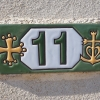##### Age 11 to 16Challenge Level

Is there a quick and easy way to calculate the sum of the first 100 odd numbers?### More Number Sandwiches

##### Age 11 to 16Challenge Level

When is it impossible to make number sandwiches?### Where Are the Primes?

##### Age 11 to 16Challenge Level

What can we say about all the primes which are greater than 3?### Arithmagons

##### Age 11 to 16Challenge Level

Can you find the values at the vertices when you know the values on the edges?### Three Consecutive Odd Numbers

##### Age 11 to 16Challenge Level

How many sets of three consecutive odd numbers can you find, in which all three numbers are prime?### Take Three from Five

##### Age 11 to 16Challenge Level

Caroline and James pick sets of five numbers. Charlie tries to find three that add together to make a multiple of three. Can they stop him?### Marbles in a Box

##### Age 11 to 16Challenge Level

How many winning lines can you make in a three-dimensional version of noughts and crosses?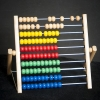### What Does it All Add up To?

##### Age 11 to 18Challenge Level

If you take four consecutive numbers and add them together, the answer will always be even. What else do you notice?### Different Products

##### Age 14 to 16Challenge Level

Take four consecutive whole numbers. Multiply the first and last numbers together. Multiply the middle pair together. What do you notice?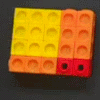##### Age 14 to 16Challenge Level

Can you find out what is special about the dimensions of rectangles you can make with squares, sticks and units?### What's Possible?

##### Age 14 to 16Challenge Level

Many numbers can be expressed as the difference of two perfect squares. What do you notice about the numbers you CANNOT make?### Painted Cube

##### Age 14 to 16Challenge Level

Imagine a large cube made from small red cubes being dropped into a pot of yellow paint. How many of the small cubes will have yellow paint on their faces?### Attractive Tablecloths

##### Age 14 to 16Challenge Level

Charlie likes tablecloths that use as many colours as possible, but insists that his tablecloths have some symmetry. Can you work out how many colours he needs for different tablecloth designs?### Latin Numbers

##### Age 14 to 16Challenge Level

Can you create a Latin Square from multiples of a six digit number?### Impossible Sums

##### Age 14 to 18Challenge Level

Which numbers cannot be written as the sum of two or more consecutive numbers?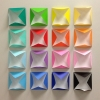### Difference of Odd Squares

##### Age 14 to 18Challenge Level

$40$ can be written as $7^2 - 3^2.$ Which other numbers can be written as the difference of squares of odd numbers?### Integration Matcher

##### Age 16 to 18Challenge Level

Can you match the charts of these functions to the charts of their integrals?### Polynomial Interpolation

##### Age 16 to 18Challenge Level

Can you fit polynomials through these points?### Prime Sequences

##### Age 16 to 18Challenge Level

This group tasks allows you to search for arithmetic progressions in the prime numbers. How many of the challenges will you discover for yourself?### Stats Statements

##### Age 16 to 18Challenge Level

Are these statistical statements sometimes, always or never true? Or it is impossible to say?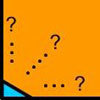### Impossible Square?

##### Age 16 to 18Challenge Level

Can you make a square from these triangles?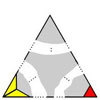### Impossible Triangles?

##### Age 16 to 18Challenge Level

Which of these triangular jigsaws are impossible to finish?### What's a Group?

##### Age 16 to 18Challenge Level

Explore the properties of some groups such as: The set of all real numbers excluding -1 together with the operation x*y = xy + x + y. Find the identity and the inverse of the element x.### Dodgy Proofs

##### Age 16 to 18Challenge Level

These proofs are wrong. Can you see why?